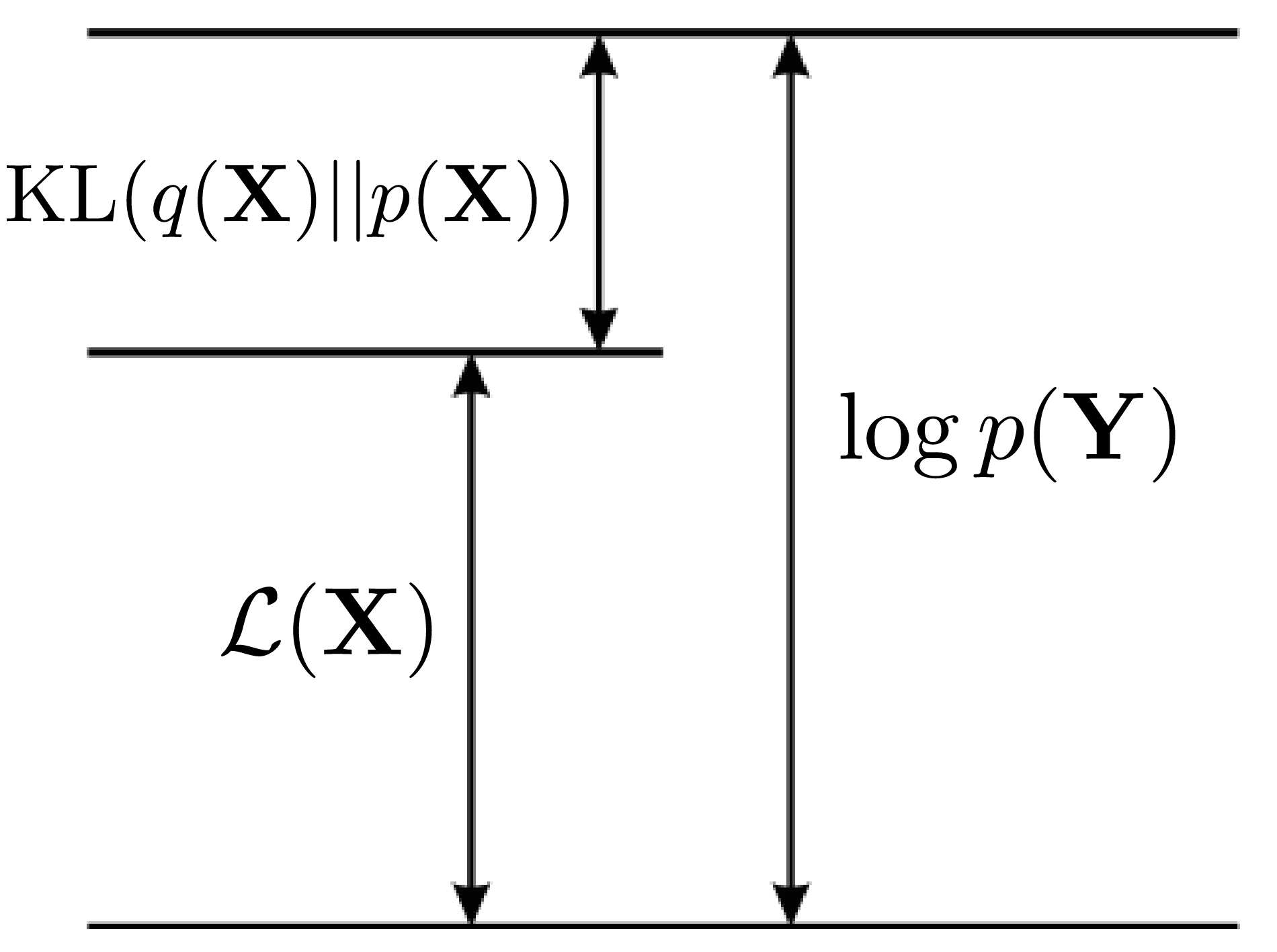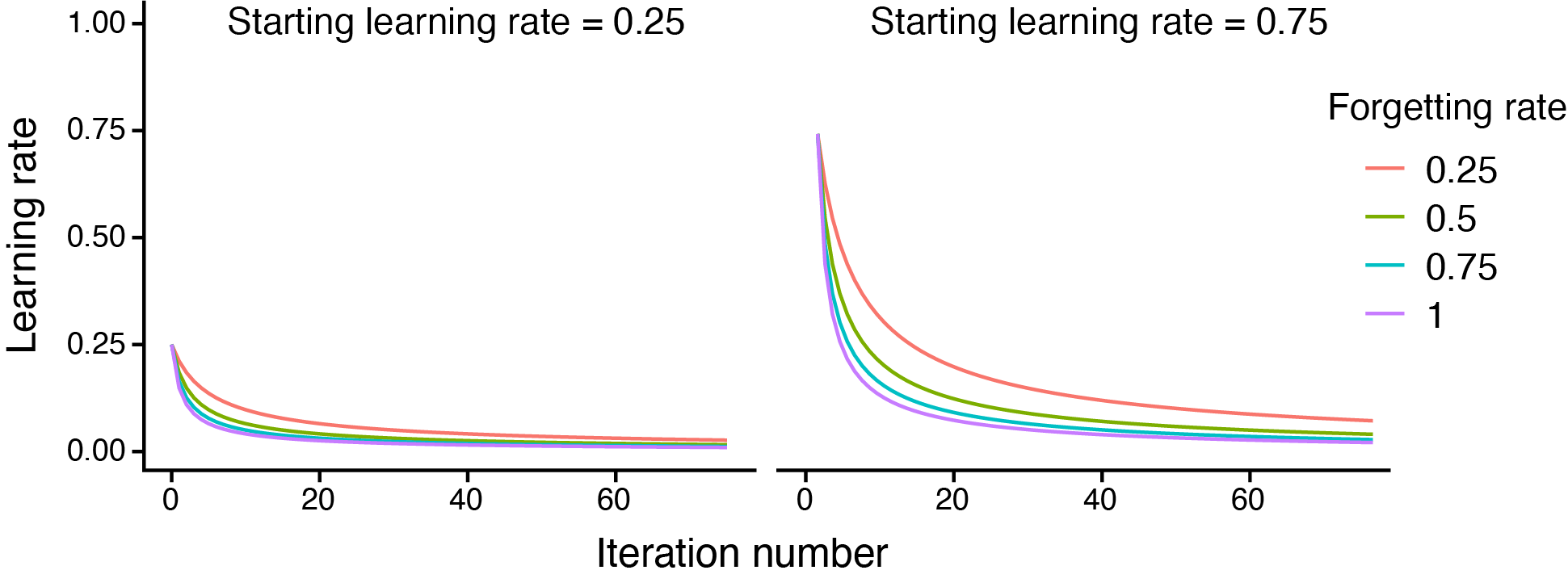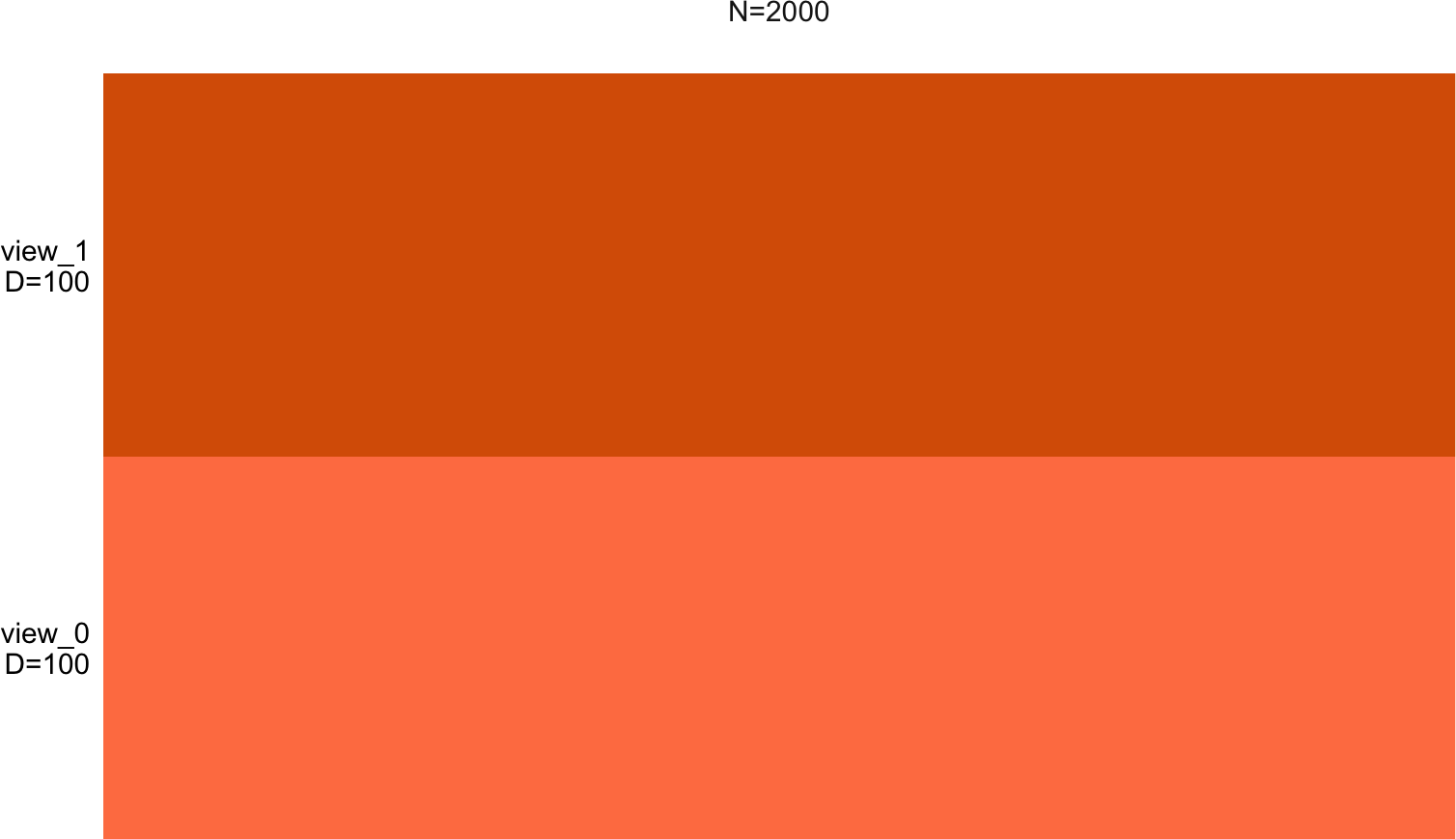The size of biological datasets is rapidly increasing, particularly in the field of single cell sequencing, with some studies reporting more than a milion cells. In the original MOFA model, inference was performed using variational Bayes. While this framework is typically faster than sampling-based Monte Carlo approaches, it becomes prohibitively slow with very large datasets, hence motivating the development of a more efficient inference schemes. For this purpose, we derived a stochastic version of the variational inference algorithm.

# 1 Theory

## 1.1 What is variational Bayes inference?

In the Bayesian probabilistic framework, the parameters $$\mathcal{L}$$ are treated as random unobserved variables and we aim to obtain probability distributions for them. To do so, prior beliefs are introduced into the model by specifying a prior probability distribution $$p(\mathcal{L})$$. Then, using Bayes’ theorem , the prior hypothesis is updated based on the observed data $${\bf Y}$$ by means of the likelihood $$p({\bf Y}|\mathcal{L})$$ function, which yields a posterior distribution over the parameters: $p(\mathcal{L}|{\bf Y}) = \frac{p({\bf Y}|\mathcal{L}) p(\mathcal{L})}{p({\bf Y})}$ where $$p({\bf Y})$$ is a constant term called the marginal likelihood, or model evidence.

The central task in Bayesian inference is the direct evaluation of the posterior distributions. In sufficiently complex models, closed-form solutions are not available and one has to resort to approximation schemes. Arguably the most commonly used approach by Bayesian purists is Markov Chain Monte Carlo (MCMC) sampling, which has the appealing property of generating exact results at the asymptotic limit of infinite computational resources. However, in practice, sampling approaches are computationally demanding and suffer from limited scalability to large data sets.

Variational inference is a deterministic approach that is based on analytical approximations to the posterior distribution, which often lead to biased results. Yet, given the appropriate settings, these approaches yield remarkably accurate results and can scale to large data sets.

In variational inference the true (but intractable) posterior distribution $$p({\bf X}|{\bf Y})$$ is approximated by a simpler (variational) distribution $$q({\bf X}|\mathcal{L})$$ where $$\mathcal{L}$$ are the corresponding parameters. The parameters, which we will omit from the notation, need to be tuned to obtain the closest approximation to the true posterior.\ The distance between the true distribution and the variational distribution is calculated using the KL divergence: ${\rm KL}(q({\bf X})||p({\bf X}|{\bf Y})) = - \int_z q({\bf X}) \log \frac{p({\bf X}|{\bf Y})}{q({\bf X})}$ Note that the KL divergence is not a proper distance metric, as it is not symmetric. In fact, using the reverse KL divergence $${\rm KL}(q({\bf X})||p({\bf X}|{\bf Y}))$$ defines a different inference framework called expectation propagation .

If we allow any possible choice of $$q({\bf X})$$, then the minimum of this function occurs when $$q({\bf X})$$ equals the true posterior distribution $$p({\bf X}|{\bf Y})$$. Nevertheless, since the true posterior is intractable to compute, this does not lead to any simplification of the problem. Instead, it is necessary to consider a restricted family of distributions $$q({\bf X})$$ that are tractable to compute and subsequently seek the member of this family for which the KL divergence is minimised.

Doing some calculus it can be shown that the KL divergence $${\rm KL}(q({\bf X})||p({\bf X}|{\bf Y}))$$ is the difference between the log of the marginal probability of the observations $$\log({\bf Y})$$ and a term $$\mathcal{L}({\bf X})$$ that is typically called the Evidence Lower Bound (ELBO): ${\rm KL}(q({\bf X})||p({\bf X}|{\bf Y})) = \log({\bf X}) - \mathcal{L}({\bf X})$ Hence, minimising the KL divergence is equivalent to maximising $$\mathcal{L}({\bf X})$$ : \begin{align} \label{eq_elbo1} \begin{split} \mathcal{L}({\bf X}) &= \int q({\bf X}) \Big( \log \frac{p({\bf X}|{\bf Y})}{q({\bf X})} + \log p({\bf Y}) \Big) d{\bf X}\\ %&= \int \Big( q(\bfX) \log \frac{p(\bfX|\bfY)}{q(\bfX)} + q(\bfX)\log p(\bfY) \Big) d\bfX\\ %&= \E_q [\log p(\bfX|\bfY)] - \E_q [\log q(\bfX)] + \E_q [\log p(\bfY)] \\ &= \mathbb{E}_q [\log p({\bf X},{\bf Y})] - \mathbb{E}_q [\log q({\bf X})] \end{split} \end{align} The first term is the expectation of the log joint probability distribution with respect to the variational distribution. The second term is the entropy of the variational distribution. Importantly, given a simple parametric form of $$q({\bf X})$$, each of the terms in can be computed in closed form.\

In conclusion, variational learning involves minimising the KL divergence between $$q({\bf X})$$ and $$p({\bf X}|{\bf Y})$$ by instead maximising $$\mathcal{L}({\bf X})$$ with respect to the distribution $$q({\bf X})$$. The following image summarises the general picture of variational learning (TO-DO):The next step is how to define $$q({\bf X})$$, but we will stop the introduction to variational inference here. If the reader is interested we suggest the following resources: XXX

## 1.2 How does stochastic variational inference (SVI) works?

In this section we will provide the intuition behind SVI. For a detailed mathematical derivation we refer the reader to the appendix of the MOFA+ paper.

The aim of VI is to maximise the ELBO of the model. This leads to an iterative algorithm that can be reformulated as a gradient ascent problem.
Just as a reminder, gradient ascent is a common first-order optimization algorithm for finding the maximum of a function. It works iteratively by taking steps proportional to the gradient of the function evaluated at each iteration. Formally, for a differentiable function $$F(x)$$, the iterative scheme of gradient ascent is: ${\bf x}^{(t+1)} = {\bf x}^{(t)} + \rho^{(t)} \nabla F({\bf x}^{(t)})$ At each iteration, the gradient $$\nabla F$$ is re-evaluated and a step is performed towards its direction. The step size is controlled by $$\rho^{(t)}$$, a parameter called the learning rate, which is typically adjusted at each iteration.

Gradient ascent is appealing because of its simplicity, but it becomes prohibitively slow with large datasets, mainly because of the computational cost (both in terms of time and memory) associated with the iterative calculation of gradients.
A fast approximation of the gradient $$\hat{\nabla} F$$ can be calculated using a random subset of the data (a batch, here is where the stochasticity is introduced). Formally, as in standard gradient ascent, the iterative training schedule proceeds by taking steps of size $$\rho$$ in the direction of the approximate gradient $$\hat{\nabla}F$$: ${\bf x}^{(t+1)} = {\bf x}^{(t)} + \rho^{(t)} \hat{\nabla} F({\bf x}^{(t)})$

There is a lot more technicalities missing, but this is sufficient to get the intuition behind the SVI algorithm.

### 1.2.1 Hyperparameters

Stochastic variational inference algorithm has three hyperparameters:

*Batch size**: controls the fraction of samples that are used to compute the gradients at each iteration. A trade-off exists where high batch sizes lead to a more precise estimate of the gradient, but are more computationally expensive to calculate.

*Learning rate**: controls the step size in the direction of the gradient, with high learning rates leading to higher step sizes. To ensure proper convergence, the learning rate has to be decayed during training by a pre-defined function.

*Forgetting rate**: controls the decay of the learning rate, with large values leading to faster decays.

The function that we use to decay the learning rate is: $\rho^{(t)} = \frac{\rho^0}{(1 + \kappa t)^{3/4}}$ where $$\rho^{(t)}$$ is the learning rate at iteration $$t$$, $$\rho^{(0)}$$ is the starting learning rate, and $$\kappa$$ is the forgetting rate which controls the rate of decay. The following figure shows the effect of varying the two hyperparameters.# 2 Example

library(MOFA2)
library(data.table)
library(ggplot2)

## 2.2 (Optional) set up reticulate connection with Python

# reticulate::use_python("/Users/ricard/anaconda3/envs/base_new/bin/python", required = T)

Load data in long data.frame format

file = "ftp://ftp.ebi.ac.uk/pub/databases/mofa/stochastic_vignette/data.txt.gz"

# Let's ignore groups
data[,group:=NULL]

## 2.4 Create MOFA object

Create MOFA object

MOFAobject <- create_mofa(data)
## Creating MOFA object from a data.frame...

Visualise the data structure

plot_data_overview(MOFAobject)Define model options

model_opts <- get_default_model_options(MOFAobject)

# the true number of factors for this data set is K=5
model_opts$num_factors <- 10 Define train options train_opts <- get_default_training_options(MOFAobject) # set stochastic to TRUE train_opts$stochastic <- TRUE

# set to TRUE if you have access to GPUs (see FAQ below for configuration instructions)
# train_opts$gpu_mode <- TRUE ## 2.5 Fit model using stochastic variational inference There are three options for stochastic inference that the user can modify: - batch_size: float value indicating the batch size (as a fraction of the total data set: 0.10, 0.25 or 0.50). We recommend setting batch_size to the largest value that can fit into the GPU memory. - learning_rate: starting learning rate, we recommend values from 0.75 to 1.0 - forgetting_rate: forgetting rate (we recommend values from 0.25 to 0.5) stochastic_opts <- get_default_stochastic_options(MOFAobject) Prepare the MOFA object MOFAobject <- prepare_mofa(MOFAobject, training_options = train_opts, model_options = model_opts, stochastic_options = stochastic_opts ) ## Warning in prepare_mofa(MOFAobject, training_options = train_opts, model_options = model_opts, : Stochastic inference is only recommended when you have a lot of samples (at least N>10,000)) Train the model outfile <- tempfile() MOFAmodel.svi <- run_mofa(MOFAobject, outfile) Plot ELBO (the objective function) versus iteration number # Fetch elbo elbo_per_iteration <- MOFAmodel.svi@training_stats[["elbo"]] # Prepare data.frame for plotting to.plot <- data.frame( iteration = 1:length(elbo_per_iteration), elbo = -log2(-elbo_per_iteration) ) to.plot <- to.plot[to.plot$iteration>5,]

ggplot(to.plot, aes(x=iteration, y=elbo)) +
geom_line() +
labs(x="Iteration", y="ELBO (the higher the better)") +
theme_classic()